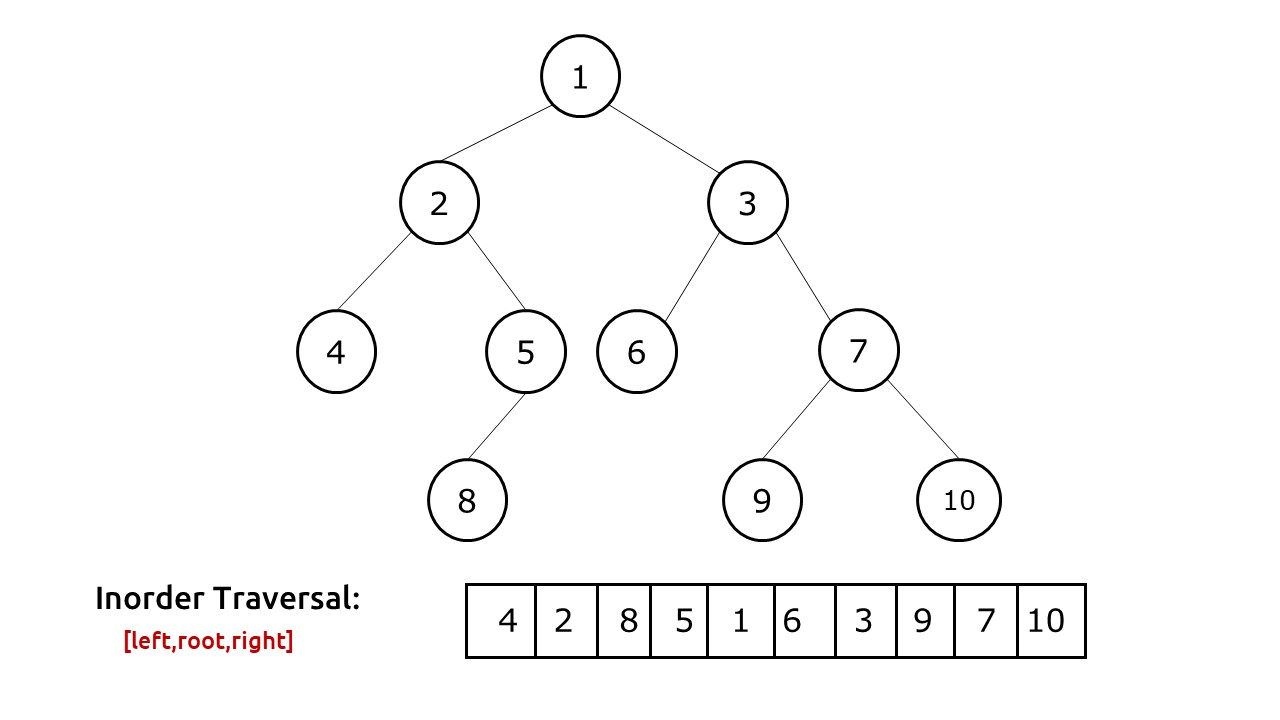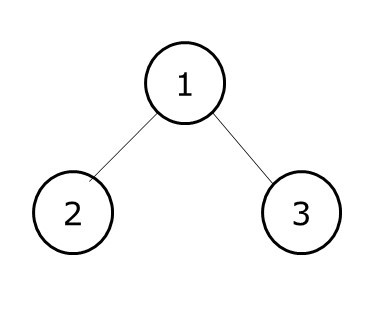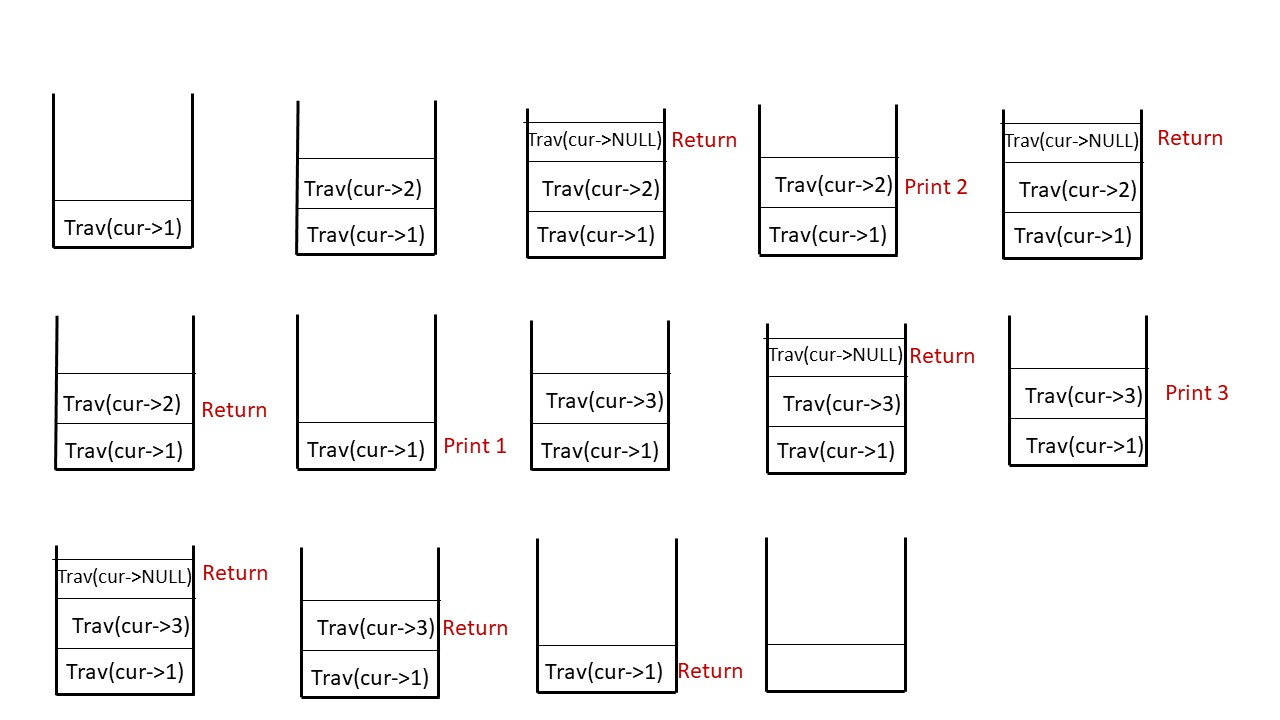# Inorder Traversal of Binary Tree

Problem Statement: Given a Binary Tree. Find and print the inorder traversal of Binary Tree.

Examples:

```Input:Output:  The inOrder Traversal is : 4 2 8 5 1 6 3 9 7 10 ```

Disclaimer: Don’t jump directly to the solution, try it out yourself first.

### Solution  [Iterative]:

Intuition: In inorder traversal, the tree is traversed in this way: root, left, right. We first visit the left child, after returning from it we print the current node value, then we visit the right child. The fundamental problem we face in this scenario is that there is no way that we can move from a child to a parent. To solve this problem, we use an explicit stack data structure. While traversing we can insert node values to the stack in such a way that we always get the next node value at the top of the stack.

Approach:

The algorithm approach can be stated as:

• We first take an explicit stack data structure and set an infinite loop.
• In every iteration we check whether our current node is pointing to NULL or not.
• If it is not pointing to null, we simply push the current value to the stack and move the current node to its left child.
• If it is pointing to NULL, we first check whether the stack is empty or not. If the stack is empty, it means that we have traversed the tree and we break out of the loop.
• If the stack is not empty, we pop the top value of the stack, print it and move the current node to its right child.

Stack is a Last-In-First-Out (LIFO) data structure, therefore when we encounter a node, we simply push it to the stack and try to find nodes on its left. When the current node points to NULL, it means that there is nothing left to traverse and we should move to the parent. This parent is always placed at the top of the stack. If the stack is empty, then we had already traversed the whole tree and should stop the execution.

Dry Run: In case you want to watch the dry run for this approach, please watch the video attached below.

Code:

## C++ Code

``````#include <bits/stdc++.h>

using namespace std;

struct node {
int data;
struct node * left, * right;
};

vector < int > inOrderTrav(node * curr) {
vector < int > inOrder;
stack < node * > s;
while (true) {
if (curr != NULL) {
s.push(curr);
curr = curr -> left;
} else {
if (s.empty()) break;
curr = s.top();
inOrder.push_back(curr -> data);
s.pop();
curr = curr -> right;
}
}
return inOrder;
}

struct node * newNode(int data) {
struct node * node = (struct node * ) malloc(sizeof(struct node));
node -> data = data;
node -> left = NULL;
node -> right = NULL;

return (node);
}

int main() {

struct node * root = newNode(1);
root -> left = newNode(2);
root -> right = newNode(3);
root -> left -> left = newNode(4);
root -> left -> right = newNode(5);
root -> left -> right -> left = newNode(8);
root -> right -> left = newNode(6);
root -> right -> right = newNode(7);
root -> right -> right -> left = newNode(9);
root -> right -> right -> right = newNode(10);

vector < int > inOrder;
inOrder = inOrderTrav(root);

cout << "The inOrder Traversal is : ";
for (int i = 0; i < inOrder.size(); i++) {
cout << inOrder[i] << " ";
}
return 0;
}``````

Output:

The inOrder Traversal is : 4 2 8 5 1 6 3 9 7 10

Time Complexity: O(N).

Reason: We are traversing N nodes and every node is visited exactly once.

Space Complexity: O(N)

Reason: In the worst case (a tree with just left children), the space complexity will be O(N).

## Java Code

``````import java.util.*;

class Node {
int data;
Node left, right;
Node(int data) {
this.data = data;
left = null;
right = null;
}
}
class TUF {
static ArrayList < Integer > inOrderTrav(Node curr) {
ArrayList < Integer > inOrder = new ArrayList < > ();
Stack < Node > s = new Stack < > ();
while (true) {
if (curr != null) {
s.push(curr);
curr = curr.left;
} else {
if (s.isEmpty()) break;
curr = s.peek();
s.pop();
curr = curr.right;
}
}
return inOrder;
}

public static void main(String args[]) {

Node root = new Node(1);
root.left = new Node(2);
root.right = new Node(3);
root.left.left = new Node(4);
root.left.right = new Node(5);
root.left.right.left = new Node(8);
root.right.left = new Node(6);
root.right.right = new Node(7);
root.right.right.left = new Node(9);
root.right.right.right = new Node(10);

ArrayList < Integer > inOrder;
inOrder = inOrderTrav(root);

System.out.println("The inOrder Traversal is : ");
for (int i = 0; i < inOrder.size(); i++) {
System.out.print(inOrder.get(i) + " ");
}
}
}``````

Output:

The inOrder Traversal is : 4 2 8 5 1 6 3 9 7 10

Time Complexity: O(N).

Reason: We are traversing N nodes and every node is visited exactly once.

Space Complexity: O(N)

Reason: In the worst case (a tree with just left children), the space complexity will be O(N).

### Solution 2 [Recursive]:

Approach: In inorder traversal, the tree is traversed in this way: left, root, right.

The algorithm approach can be stated as:

• We first recursively visit the left child and go on till we find a leaf node.
• Then we print that node value.
• Then we recursively visit the right child.
• If we encounter a node pointing to NULL, we simply return to its parent.

Explanation: It is very important to understand how recursion works behind the scenes to traverse the tree. For it we will see a simple case:Inorder traversal of this tree: 2,1,3

Initially, we pass the root node pointing to 1 to our traversal function. The algorithm steps are as follows:

• As we are doing an inorder traversal, the first thing we will do is to recursively visit the left child. We continue till the time we find a leaf node. At node 2, as there is no more left child, we print its value.
• Then we need to move back to node 1 but how do we do so? Remember that our nodes only have pointers to the children and not to the parent, therefore we can move only from parent to child and not from a child to the parent.
• The answer to this question is recursion. When we move to node 2, we call it recursively. This second function is then pushed to our call stack. We do our execution which is to visit the left child of node 2, return from it as it is NULL, print current node value and then again recursively call its right child.
• This second function will then be removed from our call stack and we will return to the first function. Then we again recursively call the function for the right child and do the execution, i.e print 1 and then visit its right child.

The call stack diagram will help to understand the recursion better.Dry Run: In case you want to watch the dry run for this approach, please watch the video attached below.

Code:

## C++ Code

``````#include <bits/stdc++.h>

using namespace std;

struct node {
int data;
struct node * left, * right;
};

void inOrderTrav(node * curr, vector < int > & inOrder) {
if (curr == NULL)
return;

inOrderTrav(curr -> left, inOrder);
inOrder.push_back(curr -> data);
inOrderTrav(curr -> right, inOrder);
}

struct node * newNode(int data) {
struct node * node = (struct node * ) malloc(sizeof(struct node));
node -> data = data;
node -> left = NULL;
node -> right = NULL;

return (node);
}

int main() {

struct node * root = newNode(1);
root -> left = newNode(2);
root -> right = newNode(3);
root -> left -> left = newNode(4);
root -> left -> right = newNode(5);
root -> left -> right -> left = newNode(8);
root -> right -> left = newNode(6);
root -> right -> right = newNode(7);
root -> right -> right -> left = newNode(9);
root -> right -> right -> right = newNode(10);

vector < int > inOrder;
inOrderTrav(root, inOrder);

cout << "The inOrder Traversal is : ";
for (int i = 0; i < inOrder.size(); i++) {
cout << inOrder[i] << " ";
}
return 0;
}
``````

Output:

The inOrder Traversal is : 4 2 8 5 1 6 3 9 7 10

Time Complexity: O(N).

Reason: We are traversing N nodes and every node is visited exactly once.

Space Complexity: O(N)

Reason: Space is needed for the recursion stack. In the worst case (skewed tree), space complexity can be O(N).

## Java Code

``````import java.util.*;

class Node {
int data;
Node left, right;
Node(int data) {
this.data = data;
left = null;
right = null;
}
}
class TUF {

static void inOrderTrav(Node curr, ArrayList < Integer > inOrder) {
if (curr == null)
return;

inOrderTrav(curr.left, inOrder);
inOrderTrav(curr.right, inOrder);
}

public static void main(String args[]) {

Node root = new Node(1);
root.left = new Node(2);
root.right = new Node(3);
root.left.left = new Node(4);
root.left.right = new Node(5);
root.left.right.left = new Node(8);
root.right.left = new Node(6);
root.right.right = new Node(7);
root.right.right.left = new Node(9);
root.right.right.right = new Node(10);

ArrayList < Integer > inOrder = new ArrayList < > ();
inOrderTrav(root, inOrder);

System.out.println("The inOrder Traversal is : ");
for (int i = 0; i < inOrder.size(); i++) {
System.out.print(inOrder.get(i) + " ");
}
}
}``````

Output:

The inOrder Traversal is : 4 2 8 5 1 6 3 9 7 10

Time Complexity: O(N).

Reason: We are traversing N nodes and every node is visited exactly once.

Space Complexity: O(N)

Reason: Space is needed for the recursion stack. In the worst case (skewed tree), space complexity can be O(N).